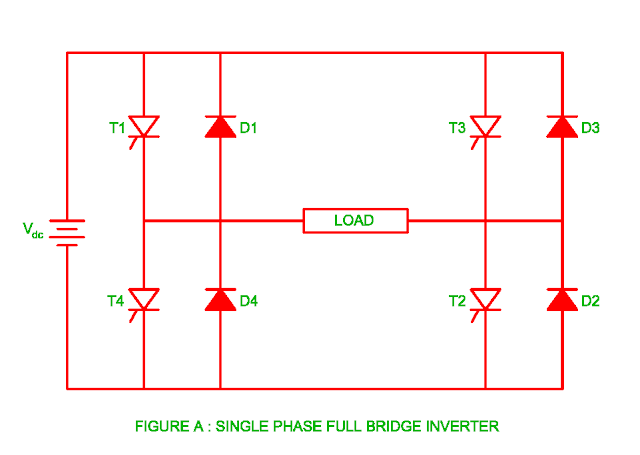## 19 June 2017

### Single Phase Full Bridge Inverter

• The power circuit diagram for the single phase full bridge inverter is shown in the figure A.
• The SCR triggering circuit and commutation circuit is not shown for simplicity. There are two wire DC supply source, four freewheeling diodes and four SCRs used in this inverter.• The SCR T1 and SCR T2 are turned on at same time whose frequency is f = 1/T.
• Similarly the SCR T3 and SCR T4 are turned on simultaneously.
• There is 1800 phase difference between positive group of SCRs ( SCR T1 and SCR T2 ) and negative group of SCRs ( SCR T3 and SCRT4 ).

The working of single phase full bridge inverter is explain as follows

### Mode 1

• The load voltage becomes to + Vdc when SCR T1 and SCR T2 are turned on at same time.
• Therefore the load current flows through path (+)Vdc – SCR T1 – load – SCR T2 – Vdc(-).
•  The load current and load voltage becomes positive during this period therefore the power flows from supply to load.

### Mode 2

• The polarity of voltage across load changes due to inductive load and as soon as the SCR T1 and SCR T2 are turned off in this mode.
• As the diode D3 and D4 conduct, the load current flows through path diode D3 – (+)Vdc – Vdc(-) – diode D4.
• The power flows from load to supply during this mode.

### Mode 3

• The SCR T1 and SCRT2 are turned off whereas SCR T3 and SCR T4 are turned on during this mode.
• The voltage across load becomes – Vdc and current flows through path (+)Vdc – SCR T3 – load – SCR T4 – Vdc(-).
• The power flows through supply to load as the load voltage and load current both are negative during this period.

### Mode 4

• The polarity of voltage across load changes as the SCR T3 and SCR T4 are turned off in this mode.
• The load current flows through path diode D1 – (+)Vdc – Vdc(-) – diode D2 – load due to conduction of freewheeling diode D1 and D2.
• The power flows from load to supply as the direction of load current reverses during this mode.

You may also like :

Principle of Inverter

Voltage Source Inverter

Current Source Inverter

Step up cycloconverter

Armature Reaction in the DC Generator